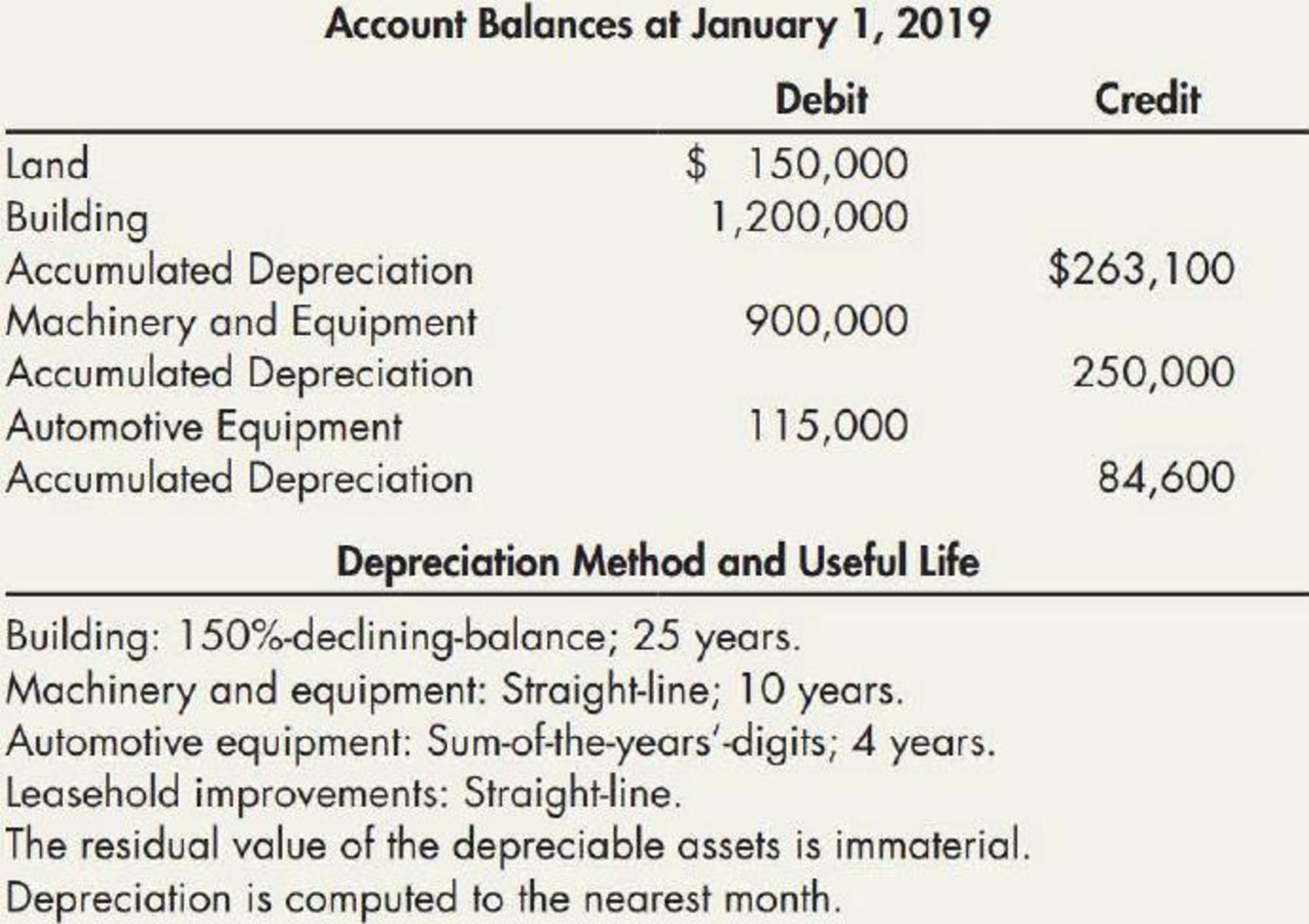Chapter 11, Problem 19P### Intermediate Accounting: Reporting...

3rd Edition
James M. Wahlen + 2 others
ISBN: 9781337788281

#### Solutions

Chapter
Section### Intermediate Accounting: Reporting...

3rd Edition
James M. Wahlen + 2 others
ISBN: 9781337788281
Textbook Problem
30 views

# Information for Blake Corporation’s property, plant, and equipment for 2019 is:Transactions during 2019 and other information were as follows: a. On January 2, 2019, Blake purchased a new car for $10,000 cash and a trade-in of a 2-year-old car with a cost of$9,000 and a book value of $2,700. The new car has a cash price of$12,000; the market value of the trade-in is not known. b. On April 1, 2019, a machine purchased for $23,000 on April 1, 2014, was destroyed by fire. Blake recovered$15,500 from its insurance company. c. On May 1, 2019, costs of $168,000 were incurred to improve leased office premises. The leasehold improvements have a useful life of 8 years. The related lease, which terminates on December 31, 2025, is renewable for an additional 6-year term. The decision to renew will be made in 2025 based on office space needs at that time. d. On July 1, 2019, machinery and equipment were purchased at a total invoice cost of$280,000; additional costs of $5,000 for freight and$25,000 for installation were incurred. e. Blake determined that the automotive equipment comprising the $115,000 balance at January 1, 2019, would have been depreciated at a total amount of$18,000 for the year ended December 31, 2019. Required: 1. For each asset classification, prepare schedules showing depredation and amortization expense, and accumulated depreciation and amortization that would appear on Blake’s income statement for the year ended December 31, 2019, and on the balance sheet at December 31, 2019, respectively. 2. Prepare a schedule showing the gain or loss from disposal of assets that would appear in Blake’s income statement for the year ended December 31,2019. 3. Prepare the property, plant, and equipment section of Blake’s December 31, 2019, balance sheet.

1.

To determine

Prepare a schedule screening the depreciation expense, amortization expense, accumulated depreciation and amortization for each asset that would appear on the income statement and balance sheet of Company B for the year ended December 31, 2019.

Explanation

Depreciation expense: Depreciation expense is a non-cash expense, which is recorded on the income statement reflecting the consumption of economic benefits of long-term asset on account of its wear and tear or obsolesces.

Straight-line depreciation method: The depreciation method which assumes that the consumption of economic benefits of long-term asset could be distributed equally throughout the useful life of the asset is referred to as straight-line method.

Sum-of- the-years’ digits method: Sum-of-the years’ digits method determines the depreciation by multiplying the depreciable base and declining fraction.

Double-declining-balance method: The depreciation method which assumes that the consumption of economic benefits of long-term asset is high in the early years but gradually declines towards the end of its useful life is referred to as double-declining-balance method.

Prepare a schedule screening the depreciation expense, amortization expense, accumulated depreciation and amortization for each asset that would appear on the income statement and balance sheet of Company B for the year ended December 31, 2019 as follows:

Depreciation and amortization expense:

 Company B Depreciation and amortization expense For the year ended December 31, 2019 Particulars $Depreciation expense for building (1)$56,214 Depreciation expense for equipment and machinery (6) $103,775 Depreciation expense for automotive equipment (10)$21,000 Amortization expense for leasehold improvements (11) $16,800 Total depreciation and amortization expense for 2019$197,789

Table (1)

Accumulated depreciation and amortization:

 Company B Accumulated depreciation and amortization December 31, 2019 Particulars Amount Amount Accumulated depreciation-Building: Balance, January 1, 2019 $263,100 Add: Depreciation expense for 2019$56,214 Balance, December 31, 2019 $319,314 Accumulated depreciation-Machinery and equipment: Balance, January 1, 2019$250,000 Add: Depreciation expense for 2019 $103,775 Sub-total$353,775 Less: Machine destroyed by fire (12) $11,500 Balance, December 31, 2019$342,275 Accumulated depreciation-Automotive equipment: Balance, January 1, 2019 $84,600 Add: Depreciation expense for 2019$21,000 Sub-total $105,600 Less: Sold car during the year (13)$6,300 Balance, December 31, 2019 $99,300 Accumulated amortization-Leasehold improvements: Amortization for 2019$16,800 Total accumulated depreciation and amortization for 2019 $777,689 Table (2) Working note (1): Compute the straight line rate: Useful life = 25 years Depreciation rate=100%25 years×1.5=6% Working note (2): Calculate the depreciation expense of building under 150%-declining balance method. Depreciation expense=(Aquisition costAccumulated depreciation)×Depreciation Rate (1)=($1,200,000$263,100)×6100=$56,214

Working note (3):

Calculate the depreciation expense of remaining machinery under straight line method.

Depreciation expense = (Cost of acquisation Residual value)(The cost of machine destoryed by fire )Estimated useful life=($900,000$0)$23,00010 years=$87,700

Working note (4):

Calculate the depreciation expense for machine destroyed by fire under straight line method.

Depreciation expense = [(The cost of machine destoryed by fire )Estimated useful life×Depreciation incurredMonths in a year]=$23,00010 years×3 months (Januray to March)12 months=$575

Working note (5):

Calculate the depreciation expense for new machinery under straight line method.

Depreciation expense = [(Cost of machineResidual value  )Estimated useful life×Depreciation incurredMonths in a year]=$310,000$010 years×6 months (July to December)12 months=\$15,500

Working note (6):

Calculate the total depreciation expense for machinery for 2019

2.

To determine

Prepare a schedule screening the gain or loss from disposal of assets of Company B that would appear on the income statement for the year ended December 31, 2019.

3.

To determine

Prepare the balance sheet of Company B and show the property, plant and equipment section as on December 31, 2019.

### Still sussing out bartleby?

Check out a sample textbook solution.

See a sample solution

#### The Solution to Your Study Problems

Bartleby provides explanations to thousands of textbook problems written by our experts, many with advanced degrees!

Get Started

#### Find more solutions based on key concepts